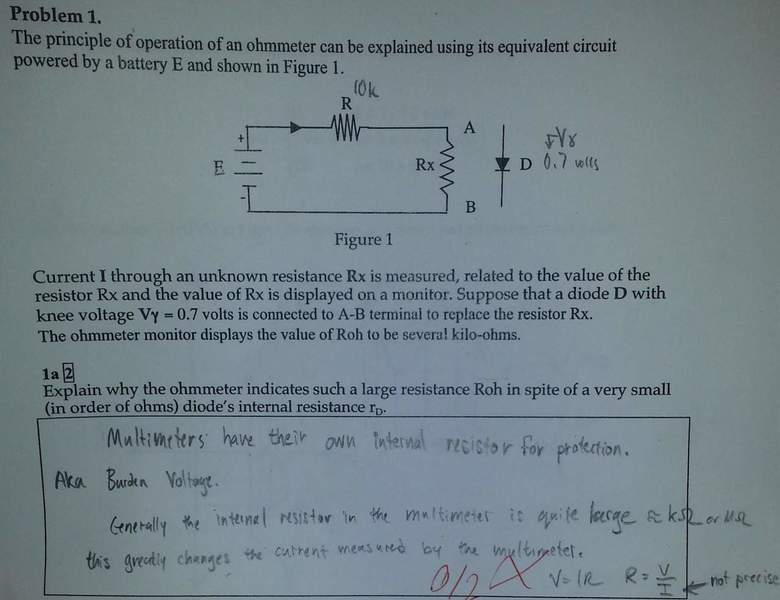# Ohmmeter & Diodes

## Homework Statement2. The attempt at a solution

So, I know that you can test a Diode with a ohmmeter.

Based off of the R you get Very large reversed biased and fairly low forward biased. But I don't know why it would show these values.

The Large one, revered biased. Kinda makes sense to me since it would have to have a large current to breakdown the diode. potentially...? I'm not quite sure really.

Since Rd is generally about 7ish Ohms, I assume both have to do with the internal current required to drive the diode.

any ideas would be helpful, THX

NascentOxygen
Staff Emeritus
Hi JeeebeZ. can you estimate the current (roughly) that the circuit will send through the diode? (To do this, you need to estimate the voltage, E.)

rude man
Homework Helper
Gold Member
For the forward direction your ohmmeter will measure V/i where i is the current it sends thru your diode and V the voltage across it. The relationship for your diode is (p-n junction assumed) i = i0exp(V/VT) where i0 = reverse saturation current and VT ~ 26 mV at room temperature. V will be around 0.7V for a Silicon and 0.3V for a Germanium diode.

From this formula you can see that the current for any negative V (reverse voltage) is ~ i0 which is maybe 2e-15A or 2e-12 mA. This is called the leakage current since for an ideal diode the reverse-voltage current = 0.

However, when you hook up the reverse connection so that V is negative, depending on your battery voltage used for the high - resistance settings, the diode MIGHT "break down" and conduct a lot more than i0/SUB]. This is unlikely; for most diodes like the popular 1N4148 the breakdown voltage is about 100V.

Referring to the question, what is di/dV which is 1/(dynamic resistance), vs. i/V which is 1/what the ohmmeter measures?

Last edited: